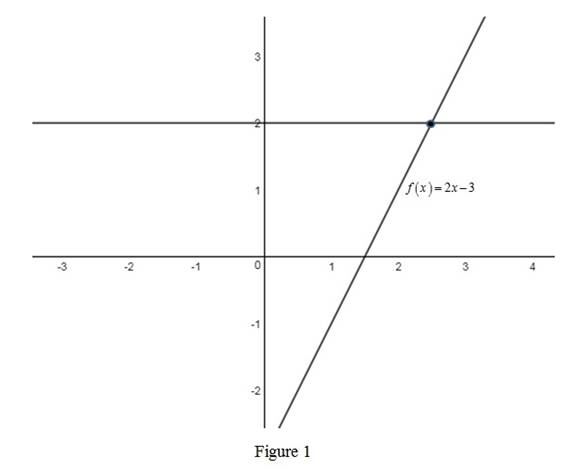# Whether the function f ( x ) = 2 x − 3 is one-to-one.### Single Variable Calculus: Concepts...

4th Edition
James Stewart
Publisher: Cengage Learning
ISBN: 9781337687805### Single Variable Calculus: Concepts...

4th Edition
James Stewart
Publisher: Cengage Learning
ISBN: 9781337687805

#### Solutions

Chapter 1.6, Problem 9E
To determine

## Whether the function f(x)=2x−3 is one-to-one.

Expert Solution

The function f(x)=2x3 is one-to one.

### Explanation of Solution

Use horizontal line test to determine whether the function f(x)=2x3 is one-to-one.

Horizontal line test states that the graph of the function is one-to-one function if and only if a horizontal line intersects the graph exactly once.

Sketch the graph of the function f(x)=2x3 and perform the horizontal line test as shown below in Figure 1.From Figure 1, it is observed that the horizontal line intersects the curve exactly at once which means it passes the horizontal line test. Therefore, the function f(x)=2x3 is one-to-one.

### Have a homework question?

Subscribe to bartleby learn! Ask subject matter experts 30 homework questions each month. Plus, you’ll have access to millions of step-by-step textbook answers!